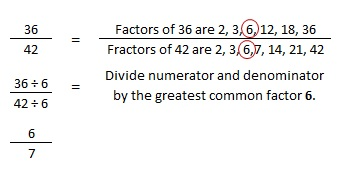## Simplifying Fraction is not as hard as You Think!

Sometimes we may have some misimpression about fraction especially on how to simplify it. We may even wonder why we have to simplify it. Can we even do the calculation without the additional steps of simplifying it? Some may think that it is really difficult and it is true. It is difficult when we don’t know how to do it. It is difficult when we don’t have the necessary knowledge to begin with. But in this post, I will show some of the techniques on how to simplify fraction easily so that you will believe that simplifying fraction is not as hard as you think!

##### Methods I Pesonally Used

First I will show my technique on how I simplify fraction. For example, the fraction is 6/8. By looking at it, you think if both numerator and denominator are divisible by two or not. This is what I called “guessing style with two or three”. The fraction 6/8 can simplified to ¾ by dividing both by two using my “guessing style with two or three” technique. This technique is not a standard because it is some kind of a trick but it really helps most of the time. This trick is not applicable to fraction that can only be divided by five or seven. For this technique, when a fraction is not divisible by two then you do it with three. When the fraction is divided by two and the result can still be divided by two or three, continue the process until it cannot be divided anymore. Then that will be the simplest form of fraction.

My second technique is an ancient method that has already been used by merchants during early Egyptian civilization. Because of this complicated process, this method has been rarely used. This method of simplifying fraction is using two numbers which are big and small. Of course this method is used on my fraction calculator on this website index page because its complicated process is suitable for programming side. The numbers used are both the numerator and denominator. Whichever bigger is designated as the big number and the smaller is designated as the smaller number. The process is to divide the bigger number by the smaller number. If the remainder is not zero and it is smaller than the smaller number, then the smaller number will become the bigger number and the remainder will become the smaller number. But if the remainder is bigger than the smaller number, the remainder will become the bigger number while the smaller number remains the same. Repeat the whole process until there is no more remainder or the remainder is zero. If the remainder is zero, the smaller number will become the common factor. Divide both the numerator and the denominator by the common factor. The result is the simplest form of the fraction.

##### Simplifying Fraction Standard Methods

Now we will discuss about the standard methods of simplifying fractions. Standard methods mean that they are universally accepted and are used in schools, universities, and industries in simplifying fractions. There are two standard methods to simplify fraction. These methods are explained and elaborated below.

The first method is called a repeated division method. This is done by listing all the factors of numerator and denominator. When the list is made, you will select the smallest common factor and used it to divide both the numerator and denominator. If the result is not in its simplest form you have to repeat the whole process again until there are no more factors left in the list. In the list, we omit 1 as a factor because using 1 as a factor will give the impression that the fraction is already in its simplest form. So now we will use 36/42 fraction as an example. See the whole process executed below.

The second method is called the greatest common factor method. The process of this method is the same from the first method but instead of selecting the lowest common factor and having the process repeated by two or more times, this method will select the greatest common factor instead and use it as a divisor of both numerator and denominator. The result is definitely the simplest form of the given fraction. See the picture below on how the process of this method is being done.Now that you know all the methods including my tricks on simplifying fractions, you can be sure that simplifying fraction is easy and is not as hard as you think!